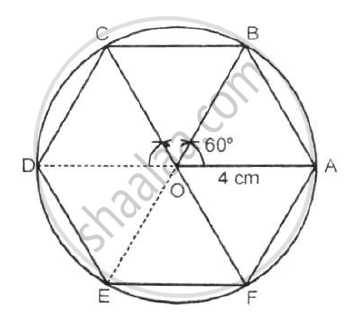Share

# Construct a Regular Hexagon of Side 4 Cm. Construct a Circle Circumscribing the Hexagon. - Mathematics

Course

#### Question

Construct a regular hexagon of side 4 cm. Construct a circle circumscribing the hexagon.

#### SolutionSteps of constructions:

1) Draw a circle of radius 4 cm with centre O.

2) Since  360^@/6 = 60^@, draw radii OA and OB, such that ∠AOB = 60°

3) Cut off arcs BC, CD, DE, EF and each equal to arc AB on given circle.

4) Join AB, BC, CD, DE, EF and FA to get required regular hexagon ABCDEF in a given circle.

Is there an error in this question or solution?